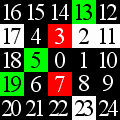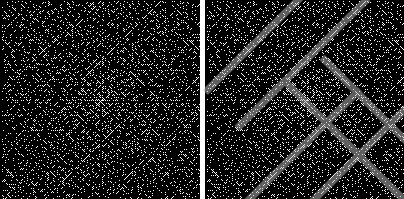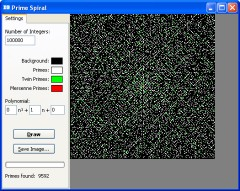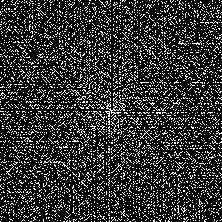# Ulam’s Prime Number Spiral

There is an infinite number of prime numbers, and yet the prime numbers themselves do not display any apparent pattern, nor does any formula exist that generates prime numbers. In fact, Legendre proved that there cannot be an algebraic function which always gives primes.However, prime numbers do exhibit a curious phenomenon when arranged in a spiral along with other consecutive integers, as in the figure to the right (in the figure, prime numbers are highlighted in white, twin primes are green, and Mersenne primes are red).

### The Phenomenon

It was first noticed by the physicist Stanisław Ulam in 1963, when he got bored in a meeting and started doodling spirals of numbers. He noticed that, if he makes a spiral of consecutive integers, and circles only the prime numbers, strange diagonal “lines” of prime numbers emerge.

This is quite surprising, since we would intuitively expect a random distribution of prime numbers. However, these diagonal segments occur on an impressively large scale, and arbitrarily far from the center of the spiral. The following image is a spiral containing about 4000 primes, and next to it is the same image with some of the diagonal paths highlighted.### Application

To explore this phenomenon on a large scale, I wrote a small program that generates arbitrarily large spirals, with configurable coloring and other options.

Download the program! (Or browse the source code on GitHub)The program generates a spiral based on the total number of integers that you specify. It also allows you to specify the colors to use for the background, prime numbers, twin primes, and Mersenne primes.

In addition, the program allows you to use a custom polynomial (up to degree-2) for generating the spiral. By default, the polynomial is set to$f(n) = n$
so the spiral will have the normal sequence 0, 1, 2, 3, etc. However, as an example, suppose you want the spiral to consist only of odd integers (1, 3, 5, 7, etc). You can simply set the polynomial to be$f(n) = 2n + 1$by writing “2” in the text box next to “n“, and “1” in the last of the three text boxes. The image to the right shows a spiral constructed from odd integers only. Notice the prominent “patterns,” this time extending vertically and horizontally.

### Conclusions

Ultimately, all that these patterns show is that certain polynomials are more “likely” to generate prime numbers than others. In fact, we can speculate that these kinds of patterns would emerge if we arrange the integers in any ordered design, not just a spiral. Even if we arrange the integers in a simple table, we would still see occasional “streaks” of prime numbers similar to the ones seen in the spiral.

The existence of “prime-generating” polynomials was known since the time of Euler, who discovered a polynomial that gives 40 consecutive prime numbers, namely
$$f(n) = n^2 – n + 41$$
The reason why some polynomials generate more primes than others is still not known.

### Extreme Spirals

Using my program, you can generate extremely large spirals, limited only by the amount of memory on your computer. Here are some fairly large ones that I generated: